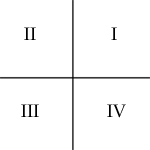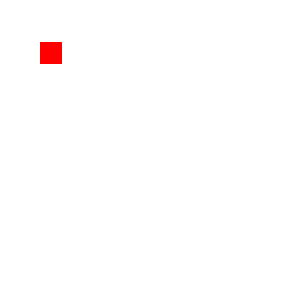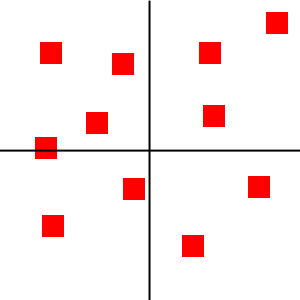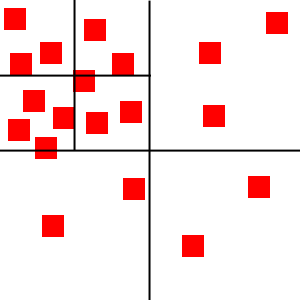# 2D空间中使用Quadtree四叉树进行碰撞检测优化

【注：这里算法的实现使用的Java语言，但算法和语言没有关系，理解了其中的原理可以应用到各种碰撞检测场景中。】

## 介绍

width="600" height="450" src="https://www.youtube.com/embed/_viW2ZUMlvo" allowfullscreen="">

## 关于四叉树## 四叉树的实现

【译者注】：这里的Java实现中坐标系原点位于左上角，物体的锚点也位于左上角。

Quadtree.java

public class Quadtree {

private int MAX_OBJECTS = 10;
private int MAX_LEVELS = 5;

private int level;        // 子节点深度
private List objects;     // 物体数组
private Rectangle bounds; // 区域边界
private Quadtree[] nodes; // 四个子节点

/*
* 构造函数
*/
public Quadtree(int pLevel, Rectangle pBounds) {
level = pLevel;
objects = new ArrayList();
bounds = pBounds;
nodes = new Quadtree;
}
}

Quadtree类的结构很清楚：

• MAX_OBJECTS：定义的是一个区域节点在被划分之前能够拥有节点的最大数量；
• MAX_LEVELS：定义的是子节点的最大深度；
• level：指的是当前节点的深度，对于自身来说level为0；
• bounds：指的是当前节点所占的2d空间；
• nodes：四个子节点。

clear方法递归清除所有节点所拥有的物体：

/*
* 清空四叉树
*/
public void clear() {
objects.clear();

for (int i = 0; i < nodes.length; i++) {
if (nodes[i] != null) {
nodes[i].clear();
nodes[i] = null;
}
}
}

split函数将当前节点平均分成四个子节点，并用计算好的新节点数据初始化四个子节点：

/*
* 将一个节点分成四个子节点（实际是添加四个子节点）
*/
private void split() {
int subWidth = (int)(bounds.getWidth() / 2);
int subHeight = (int)(bounds.getHeight() / 2);
int x = (int)bounds.getX();
int y = (int)bounds.getY();

nodes = new Quadtree(level+1, new Rectangle(x + subWidth, y, subWidth, subHeight));
nodes = new Quadtree(level+1, new Rectangle(x, y, subWidth, subHeight));
nodes = new Quadtree(level+1, new Rectangle(x, y + subHeight, subWidth, subHeight));
nodes = new Quadtree(level+1, new Rectangle(x + subWidth, y + subHeight, subWidth, subHeight));
}

getIndex函数判断物体属于父节点还是子节点，以及属于哪一个子节点：

/*
* 用于判断物体属于哪个子节点
* -1指的是当前节点可能在子节点之间的边界上不属于四个子节点而还是属于父节点
*/

private int getIndex(Rectangle pRect) {
int index = -1;
// 中线
double verticalMidpoint = bounds.getX() + (bounds.getWidth() / 2);
double horizontalMidpoint = bounds.getY() + (bounds.getHeight() / 2);

// 物体完全位于上面两个节点所在区域
boolean topQuadrant = (pRect.getY() < horizontalMidpoint && pRect.getY() + pRect.getHeight() < horizontalMidpoint);
// 物体完全位于下面两个节点所在区域
boolean bottomQuadrant = (pRect.getY() > horizontalMidpoint);

// 物体完全位于左面两个节点所在区域
if (pRect.getX() < verticalMidpoint && pRect.getX() + pRect.getWidth() < verticalMidpoint) {
if (topQuadrant) {
index = 1; // 处于左上节点
}
else if (bottomQuadrant) {
index = 2; // 处于左下节点
}
}
// 物体完全位于右面两个节点所在区域
else if (pRect.getX() > verticalMidpoint) {
if (topQuadrant) {
index = 0; // 处于右上节点
}
else if (bottomQuadrant) {
index = 3; // 处于右下节点
}
}

return index;
}

insert函数往四叉树中添加物体，如果物体可以分给子节点则分给子节点，否则就留给父节点了，父节点物体超出容量后如果没分裂的话就分裂从而将物体分给子节点：

/*
* 将物体插入四叉树
* 如果当前节点的物体个数超出容量了就将该节点分裂成四个从而让多数节点分给子节点
*/
public void insert(Rectangle pRect) {

// 插入到子节点
if (nodes != null) {
int index = getIndex(pRect);

if (index != -1) {
nodes[index].insert(pRect);

return;
}
}

// 还没分裂或者插入到子节点失败，只好留给父节点了
objects.add(pRect);

// 超容量后如果没有分裂则分裂
if (objects.size() > MAX_OBJECTS && level < MAX_LEVELS) {
if (nodes == null) {
split();
}
// 分裂后要将父节点的物体分给子节点们
int i = 0;
while (i < objects.size()) {
int index = getIndex(objects.get(i));
if (index != -1) {
nodes[index].insert(objects.remove(i));
}
else {
i++;
}
}
}
}

/*
* 返回所有可能和指定物体碰撞的物体
*/
public List retrieve(List returnObjects, Rectangle pRect) {
int index = getIndex(pRect);
if (index != -1 && nodes != null) {
nodes[index].retrieve(returnObjects, pRect);
}

returnObjects.addAll(objects);

return returnObjects;
}

## 应用于2d碰撞检测

Quadtree quad = new Quadtree(0, new Rectangle(0,0,600,600));

quad.clear();
for (int i = 0; i < allObjects.size(); i++) {
quad.insert(allObjects.get(i));
}

List returnObjects = new ArrayList();
for (int i = 0; i < allObjects.size(); i++) {
returnObjects.clear();
quad.retrieve(returnObjects, objects.get(i));

for (int x = 0; x < returnObjects.size(); x++) {
// 使用合适的碰撞检测算法和每一个可能碰撞的物体进行碰撞检测...
}
}

## 结论

06-0908-04
08-201万+
09-121130
07-121013
03-141965
06-0212
07-262984
08-08
06-09
12-1787
12-0674
06-163085
06-151万+
06-132万+
©️2020 CSDN 皮肤主题: 点我我会动 设计师:白松林点击重新获取扫码支付1.余额是钱包充值的虚拟货币，按照1:1的比例进行支付金额的抵扣。
2.余额无法直接购买下载，可以购买VIP、C币套餐、付费专栏及课程。余额充值